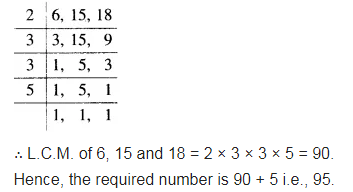# Find the least number which when divided by 6, 15 and 18 leave remainder 5 in each case

Find the least number which when divided by 6, 15 and 18 leave remainder 5 in each case.